Machine Learning Newsletter

# Supervised Learning¶

Supervised Learning is the most commonly referred/mentioned/researched/published subfield in the machine learning. Unsurprisingly, all of the predictive algorithms fall in to this category. Its premise is quite simple, when you provide a labeled dataset to the algorithm(training set), it will predict unseen dataset's (test set) output variables, whether be it a discrete label(classification) or a continuous value(regression). The learning function(regressor or classifier) will first fit to the training set and then try to predict the dataset that it has never seen, which Scikit-Learn follows these two steps literally. More on this later. First, what is the problem that we are trying to solve?

## Classification¶

#### Dataset¶

Dataset is from a telecom service provider where they have the service usage(international plan, voicemail plan, usage in daytime, usage in evenings and nights and so on) and basic demographic information(state and area code) of the user. For labels, I have a single data point whether the customer is churned out or not. Dataset is from a competititon that CrowdAnalytics did I believe and the original contest text is given in below.

#### Original Dataset Explanation¶

Churn (loss of customers to competition) is a problem for telecom companies because it is more expensive to acquire a new customer than to keep your existing one from leaving. This contest is about enabling churn reduction using analytics.

The Business Pain:

Most telecom companies suffer from voluntary churn. Churn rate has strong impact on the life time value of the customer because it affects the length of service and the future revenue of the company. For example if a company has 25% churn rate then the average customer lifetime is 4 years; similarly a company with a churn rate of 50%, has an average customer lifetime of 2 years. It is estimated that 75 percent of the 17 to 20 million subscribers signing up with a new wireless carrier every year are coming from another wireless provider, which means they are churners. Telecom companies spend hundreds of dollars to acquire a new customer and when that customer leaves, the company not only loses the future revenue from that customer but also the resources spend to acquire that customer. Churn erodes profitability.

Steps that have been adopted by telecom companies so far:

Telecom companies have used two approaches to address churn - (a) Untargeted approach and (b) Targeted approach. The untargeted approach relies on superior product and mass advertising to increase brand loyalty and thus retain customers. The targeted approach relies on identifying customers who are likely to churn, and provide suitable intervention to encourage them to stay.

Role of predictive modeling:

In the targeted approach the company tries to identify in advance customers who are likely to churn. The company then targets those customers with special programs or incentives. This approach can bring in huge loss for a company, if churn predictions are inaccurate, because then firms are wasting incentive money on customers who would have stayed anyway. There are numerous predictive modeling techniques for predicting customer churn. These vary in terms of statistical technique (e.g., neural nets versus logistic regression versus survival analysis), and variable selection method (e.g., theory versus stepwise selection).

Objective of this Contest:

The objective of this contest is to predict customer churn. We are providing you a public dataset that has customer usage pattern and if the customer has churned or not. We expect you to develop an algorithm to predict the churn score based on usage pattern. The predictors provided are as follows:

account length international plan voice mail plan number of voice mail messages total day minutes used day calls made total day charge total evening minutes total evening calls total evening charge total night minutes total night calls total night charge total international minutes used total international calls made total international charge number customer service calls made

#### Problem Formulation¶

Customer Churn: losing/attrition of the customers from the company. Especially, the industries that the user acquisition is costly, it is crucially important for one company to reduce and ideally make the customer churn to 0 to sustain their recurring revenue. If you consider customer retention is always cheaper than customer acquisition and generally depends on the data of the user(usage of the service or product), it poses a great/exciting/hard problem for machine learning.

#### Explanation of Walkthrough¶

In this section, I will first walk through a classification example where I try to predict if a customer churns or not based on a variety of attributes that she has. Customer churn prevention allows companies to react preemptively to the customer and then try to provide a better experience to her. This has two advantages to the company; product gets better with the feedback that company received from the customer and as customer continues to use the product, company would not lose the customer. Customer Relation Management(CRM) tools that has the user experience and history of the user may provide a profile for the user.

In order to do so, I will first do preprocessing on the attirbutes as most of the attributes vary a lot both intra-class and inter-class changes. Then, I will try to compare different classifiers. Since the dataset has an unbalancad classes(not churned users are 6 times of churned users), it provides also a nice case for different metrics usage as well and why classification accuracy may not be a good metric to optimize in this case.

#### Binary Classification¶

This labeling schema is arguably the most common classification schema which is called binary classification as I have only two classes to predict(churn or not). Even some of the multi-class classification problems could be expressed as a binary classification schema(one vs other classes). Therefore, it is a powerful/basic classification schema that you would use approximately 83%(put some random number > 50) of your machine learning problems.

In :
%matplotlib inline

from IPython.display import Image
import matplotlib as mlp
import matplotlib.pyplot as plt
import numpy as np
import os
import pandas as pd
import sklearn

from sklearn import cross_validation
from sklearn import tree
from sklearn import svm
from sklearn import ensemble
from sklearn import neighbors
from sklearn import linear_model
from sklearn import metrics
from sklearn import preprocessing

plt.style.use('fivethirtyeight') # Good looking plots
pd.set_option('display.max_columns', None) # Display any number of columns

_DATA_DIR = 'data'
_CHURN_DATA_PATH = os.path.join(_DATA_DIR, 'churn.csv')

import seaborn as sns

In :
df = pd.read_csv(_CHURN_DATA_PATH)
df.head()

Out:
State Account Length Area Code Phone Int'l Plan VMail Plan VMail Message Day Mins Day Calls Day Charge Eve Mins Eve Calls Eve Charge Night Mins Night Calls Night Charge Intl Mins Intl Calls Intl Charge CustServ Calls Churn?
0 KS 128 415 382-4657 no yes 25 265.1 110 45.07 197.4 99 16.78 244.7 91 11.01 10.0 3 2.70 1 False.
1 OH 107 415 371-7191 no yes 26 161.6 123 27.47 195.5 103 16.62 254.4 103 11.45 13.7 3 3.70 1 False.
2 NJ 137 415 358-1921 no no 0 243.4 114 41.38 121.2 110 10.30 162.6 104 7.32 12.2 5 3.29 0 False.
3 OH 84 408 375-9999 yes no 0 299.4 71 50.90 61.9 88 5.26 196.9 89 8.86 6.6 7 1.78 2 False.
4 OK 75 415 330-6626 yes no 0 166.7 113 28.34 148.3 122 12.61 186.9 121 8.41 10.1 3 2.73 3 False.
In :
# Discreet value integer encoder
label_encoder = preprocessing.LabelEncoder()

# Get the Labels as integers
df['Churn'] = df['Churn?'] == 'True.'
y = df['Churn'].as_matrix().astype(np.int)

# State is string and we want discre integer values
df['State'] = label_encoder.fit_transform(df['State'])

# Drop the redundant columns from dataframe
df.drop(['Area Code','Phone','Churn?', 'Churn'], axis=1, inplace=True)

# Get the features as integers similar to what we did for labels(targets)
df[["Int'l Plan","VMail Plan"]] = df[["Int'l Plan","VMail Plan"]] == 'yes'

In :
print('There are {} instances for churn class and {} instances for not-churn classes.'.format(y.sum(), y.shape - y.sum()))

There are 483 instances for churn class and 2850 instances for not-churn classes.

In :
print('Ratio of churn class over all instances: {:.2f}'.format(float(y.sum()) / y.shape))

Ratio of churn class over all instances: 0.14


Kind of unbalanced data, do not you think? I will try to handle this unbalance in the cross validation.

In :
df.head()

Out:
State Account Length Int'l Plan VMail Plan VMail Message Day Mins Day Calls Day Charge Eve Mins Eve Calls Eve Charge Night Mins Night Calls Night Charge Intl Mins Intl Calls Intl Charge CustServ Calls
0 16 128 False True 25 265.1 110 45.07 197.4 99 16.78 244.7 91 11.01 10.0 3 2.70 1
1 35 107 False True 26 161.6 123 27.47 195.5 103 16.62 254.4 103 11.45 13.7 3 3.70 1
2 31 137 False False 0 243.4 114 41.38 121.2 110 10.30 162.6 104 7.32 12.2 5 3.29 0
3 35 84 True False 0 299.4 71 50.90 61.9 88 5.26 196.9 89 8.86 6.6 7 1.78 2
4 36 75 True False 0 166.7 113 28.34 148.3 122 12.61 186.9 121 8.41 10.1 3 2.73 3

After preprocessing, dataframe is ready to be represented as matrix that is amenable to Scikit Learn. I already separated the labels, so I would just convert the dataframe into a numpy matrix. Why I did not handle True and False some may ask. It turns out that booleans False and True are actually subclasses of integers in Python. If you try to do False + True in a Python REPL, you would get 1. That is because True is represented as 1 and False is represented as 0. Numpy uses this boolean information to convert the booleans into matrix. I just need to coerce astype(np.float) when I convert the pandas dataframe to numpy matrix, which I will do exactly as next step.

In :
X = df.as_matrix().astype(np.float)

In :
X

Out:
array([[  16.  ,  128.  ,    0.  , ...,    3.  ,    2.7 ,    1.  ],
[  35.  ,  107.  ,    0.  , ...,    3.  ,    3.7 ,    1.  ],
[  31.  ,  137.  ,    0.  , ...,    5.  ,    3.29,    0.  ],
...,
[  39.  ,   28.  ,    0.  , ...,    6.  ,    3.81,    2.  ],
[   6.  ,  184.  ,    1.  , ...,   10.  ,    1.35,    2.  ],
[  42.  ,   74.  ,    0.  , ...,    4.  ,    3.7 ,    0.  ]])
In :
X.shape

Out:
(3333, 18)

I have 3333 instances and has 18 dimension feature vectors for each instances.

Since the features have quite different value ranges and some of them are discrete and some of them take continuous values, I need to scale them first. Removing mean and dividing the standard deviation of features respectively. Generally, this is one of the most commonly used preprocessing step.

In :
scaler = preprocessing.StandardScaler()
X = scaler.fit_transform(X)

In :
X

Out:
array([[-0.6786493 ,  0.67648946, -0.32758048, ..., -0.60119509,
-0.0856905 , -0.42793202],
[ 0.6031696 ,  0.14906505, -0.32758048, ..., -0.60119509,
1.2411686 , -0.42793202],
[ 0.33331299,  0.9025285 , -0.32758048, ...,  0.21153386,
0.69715637, -1.1882185 ],
...,
[ 0.87302621, -1.83505538, -0.32758048, ...,  0.61789834,
1.3871231 ,  0.33235445],
[-1.35329082,  2.08295458,  3.05268496, ...,  2.24335625,
-1.87695028,  0.33235445],
[ 1.07541867, -0.67974475, -0.32758048, ..., -0.19483061,
1.2411686 , -1.1882185 ]])

After preprocessed X, I could be sure that all of the features are in in more or less in the same range. However, one may think twice if the features are not nearly uniformed distribution(for example if a variable is categorical), you may do a different preprocessing as preprocessing makes the categorical variables like continuous variables.

### Cross Validation¶

In :
cv_url = 'http://i.imgur.com/N9HZktu.png'
Image(url=cv_url)

Out:In :
def stratified_cv(X, y, clf_class, shuffle=True, n_folds=10, **kwargs):
stratified_k_fold = cross_validation.StratifiedKFold(y, n_folds=n_folds, shuffle=shuffle)
y_pred = y.copy()
for ii, jj in stratified_k_fold:
X_train, X_test = X[ii], X[jj]
y_train = y[ii]
clf = clf_class(**kwargs)
clf.fit(X_train,y_train)
y_pred[jj] = clf.predict(X_test)
return y_pred


I am using Stratified K Fold because there the classes are unbalanced. I do not want any folds to have only 1 particular class or even 1 class dominating the other one as it may create a bias in that particular fold. Stratification makes sure that the percentage of samples for each class is similar across folds(if not same). If you do not have an unbalanced problem, KFold would work fine.

In :
print('Passive Aggressive Classifier: {:.2f}'.format(metrics.accuracy_score(y, stratified_cv(X, y, linear_model.PassiveAggressiveClassifier))))
print('Gradient Boosting Classifier:  {:.2f}'.format(metrics.accuracy_score(y, stratified_cv(X, y, ensemble.GradientBoostingClassifier))))
print('Support vector machine(SVM):   {:.2f}'.format(metrics.accuracy_score(y, stratified_cv(X, y, svm.SVC))))
print('Random Forest Classifier:      {:.2f}'.format(metrics.accuracy_score(y, stratified_cv(X, y, ensemble.RandomForestClassifier))))
print('K Nearest Neighbor Classifier: {:.2f}'.format(metrics.accuracy_score(y, stratified_cv(X, y, neighbors.KNeighborsClassifier))))
print('Logistic Regression:           {:.2f}'.format(metrics.accuracy_score(y, stratified_cv(X, y, linear_model.LogisticRegression))))

Passive Aggressive Classifier: 0.82
Gradient Boosting Classifier:  0.95
Support vector machine(SVM):   0.92
Random Forest Classifier:      0.94
K Nearest Neighbor Classifier: 0.90
Logistic Regression:           0.86


Scores seem very good, but if I predicted all of the labels 0, what would I get as accuracy rate? Let's see.

In :
print('Dump Classifier: {:.2f}'.format(metrics.accuracy_score(y, [0 for ii in y.tolist()])))

Dump Classifier: 0.86


This is because again unbalanced dataset problem. Since one class is 6 times of other class. I could get fairly accurate prediction if I predict the common class. What I need to look at as a metric if the classifier is doing well is to see the errors over the classes. Confusion matrices give a perfect way to see the distribution, which I will exactly use as a next step.

### Confusion Matrices¶

If you have an unbalanced dataset problem or if you care accuracy of one class over other(maybe false-positives are not so bad for you but you definitely do not want any false negatives), then you could display the class accuracies in confusion matrices. Very common example of class preference of one to another is in medical applications. If you are trying to predict if a patient has cancer, you could get away with some false positives(patients that do not have cancer but are told to have cancers). However, you never ever want to tell a patient that has cancer that she does not have cancer(false negative). In this type of problems, not only you should be accurate but also you cannot be wrong in ne of the squares in the confusion matrix.

Human problems cannot be solved by minimizing least squares error.

Drew Conway

Luckily, scikit learn provides a 2-D matrix for the confusion matrix under metrics submodule, which I will use to build confusion matrices for visualization(heatmap).

If you are missing one tool in your machine learning workflow, search for if Scikit-Learn has an implementation. Chances are, it has.

However, the heatmap in matplotlib implementation does not allow to print the matrix on top of the heatmap with default options. Heatmap is good in order to get a sense of where the classifier is not doing well, but not so good if you get numbers(you need to print out the confusion matrix separately). In order to combine both heatmap and also the numbers, I will use seaborn(an excellent statistical visualization library for Python) where it provides even advanced formatting for the numbers.

In :
pass_agg_conf_matrix = metrics.confusion_matrix(y, stratified_cv(X, y, linear_model.PassiveAggressiveClassifier))
grad_ens_conf_matrix = metrics.confusion_matrix(y, stratified_cv(X, y, ensemble.GradientBoostingClassifier))
decision_conf_matrix = metrics.confusion_matrix(y, stratified_cv(X, y, tree.DecisionTreeClassifier))
ridge_clf_conf_matrix = metrics.confusion_matrix(y, stratified_cv(X, y, linear_model.RidgeClassifier))
svm_svc_conf_matrix = metrics.confusion_matrix(y, stratified_cv(X, y, svm.SVC))
random_forest_conf_matrix = metrics.confusion_matrix(y, stratified_cv(X, y, ensemble.RandomForestClassifier))
k_neighbors_conf_matrix = metrics.confusion_matrix(y, stratified_cv(X, y, neighbors.KNeighborsClassifier))
logistic_reg_conf_matrix = metrics.confusion_matrix(y, stratified_cv(X, y, linear_model.LogisticRegression))
dumb_conf_matrix = metrics.confusion_matrix(y, [0 for ii in y.tolist()]); # ignore the warning as they are all 0

conf_matrix = {
1: {
'matrix': pass_agg_conf_matrix,
'title': 'Passive Aggressive',
},
2: {
'matrix': grad_ens_conf_matrix,
'title': 'Gradient Boosting',
},
3: {
'matrix': decision_conf_matrix,
'title': 'Decision Tree',
},
4: {
'matrix': ridge_clf_conf_matrix,
'title': 'Ridge',
},
5: {
'matrix': svm_svc_conf_matrix,
'title': 'Support Vector Machine',
},
6: {
'matrix': random_forest_conf_matrix,
'title': 'Random Forest',
},
7: {
'matrix': k_neighbors_conf_matrix,
'title': 'K Nearest Neighbors',
},
8: {
'matrix': logistic_reg_conf_matrix,
'title': 'Logistic Regression',
},
9: {
'matrix': dumb_conf_matrix,
'title': 'Dumb',
},
}

In :
fix, ax = plt.subplots(figsize=(16, 12))
plt.suptitle('Confusion Matrix of Various Classifiers')
for ii, values in conf_matrix.items():
matrix = values['matrix']
title = values['title']
plt.subplot(3, 3, ii) # starts from 1
plt.title(title);
sns.heatmap(matrix, annot=True,  fmt='');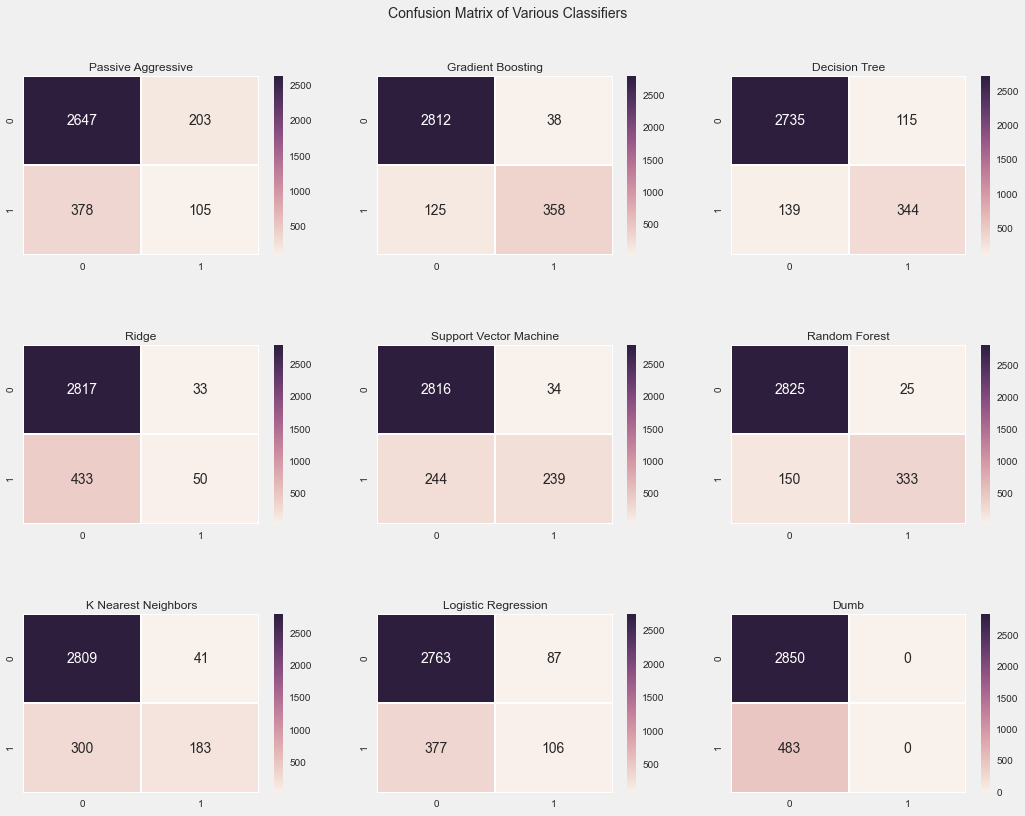Seaborn is kind of neat, huh? Gradient Boosting is indeed quite good.

### Accuracy vs. Precision vs. Recall vs. F1 Score¶

Accuracy, precision and recall are defined as follows respectively: $$accuracy = \frac{\mbox{true positives + true negatives}}{\mbox{true positives + true negatives + false positives + false negatives}}$$

$$precision = \frac{\mbox{true positives}}{\mbox{true positives + false positives}}$$$$recall = \frac{\mbox{true positives}}{\mbox{true positives + false negatives}}$$

Gradient Boosting Classifier not only is quite accurate for 0 class(not churning class) but also fairly accurate for churn class(class=1)).

Generally, for unbalanced datasets, $f_1$ score could also give a fairly accurate estimation of how well the classifier is doing considering both classes as it is the harmonic mean of precision and recall. $$f_1 = 2 \cdot \frac{pr \cdot rc}{pr + rc}$$

If we want to measure not just class distributions, but also a more abstract measures(like precision, recall or $f_1$ score), we could get those measures by using classification_report function under the metrics submodule.

In :
print('Passive Aggressive Classifier:\n {}\n'.format(metrics.classification_report(y, stratified_cv(X, y, linear_model.PassiveAggressiveClassifier))))
print('Gradient Boosting Classifier:\n {}\n'.format(metrics.classification_report(y, stratified_cv(X, y, ensemble.GradientBoostingClassifier))))
print('Support vector machine(SVM):\n {}\n'.format(metrics.classification_report(y, stratified_cv(X, y, svm.SVC))))
print('Random Forest Classifier:\n {}\n'.format(metrics.classification_report(y, stratified_cv(X, y, ensemble.RandomForestClassifier))))
print('K Nearest Neighbor Classifier:\n {}\n'.format(metrics.classification_report(y, stratified_cv(X, y, neighbors.KNeighborsClassifier))))
print('Logistic Regression:\n {}\n'.format(metrics.classification_report(y, stratified_cv(X, y, linear_model.LogisticRegression))))
print('Dump Classifier:\n {}\n'.format(metrics.classification_report(y, [0 for ii in y.tolist()]))); # ignore the warning as they are all 0

Passive Aggressive Classifier:
precision    recall  f1-score   support

0       0.88      0.93      0.90      2850
1       0.34      0.23      0.28       483

avg / total       0.80      0.82      0.81      3333

Gradient Boosting Classifier:
precision    recall  f1-score   support

0       0.96      0.99      0.97      2850
1       0.91      0.73      0.82       483

avg / total       0.95      0.95      0.95      3333

Support vector machine(SVM):
precision    recall  f1-score   support

0       0.92      0.99      0.95      2850
1       0.88      0.52      0.65       483

avg / total       0.92      0.92      0.91      3333

Random Forest Classifier:
precision    recall  f1-score   support

0       0.95      0.99      0.97      2850
1       0.92      0.69      0.79       483

avg / total       0.95      0.95      0.94      3333

K Nearest Neighbor Classifier:
precision    recall  f1-score   support

0       0.90      0.99      0.94      2850
1       0.81      0.37      0.51       483

avg / total       0.89      0.90      0.88      3333

Logistic Regression:
precision    recall  f1-score   support

0       0.88      0.97      0.92      2850
1       0.55      0.21      0.31       483

avg / total       0.83      0.86      0.83      3333

Dump Classifier:
precision    recall  f1-score   support

0       0.86      1.00      0.92      2850
1       0.00      0.00      0.00       483

avg / total       0.73      0.86      0.79      3333



Scikit-Learn provides a lot of metrics for your own evaluation. You could also build your own $f_{beta}$ score if you want to weight precision more than recall or vice versa by using fbeta_score function under metrics in Scikit Learn.

Search engines and generally information retrieval care more about precision than recall. A user could visit only so many webpages but when she visits first and second pages, she needs to see relevant/accurate results based on her query. Recall may not be very important for those cases as she is limited by time and recall may not be that important for the search results she sees.

In :
gbc = ensemble.GradientBoostingClassifier()
gbc.fit(X, y)

Out:
GradientBoostingClassifier(init=None, learning_rate=0.1, loss='deviance',
max_depth=3, max_features=None, max_leaf_nodes=None,
min_samples_leaf=1, min_samples_split=2, n_estimators=100,
random_state=None, subsample=1.0, verbose=0,
warm_start=False)
In :
# Get Feature Importance from the classifier
feature_importance = gbc.feature_importances_
# Normalize The Features
feature_importance = 100.0 * (feature_importance / feature_importance.max())
sorted_idx = np.argsort(feature_importance)
pos = np.arange(sorted_idx.shape) + .5
plt.figure(figsize=(16, 12))
plt.barh(pos, feature_importance[sorted_idx], align='center', color='#7A68A6')
plt.yticks(pos, np.asanyarray(df.columns.tolist())[sorted_idx])
plt.xlabel('Relative Importance')
plt.title('Variable Importance')
plt.show()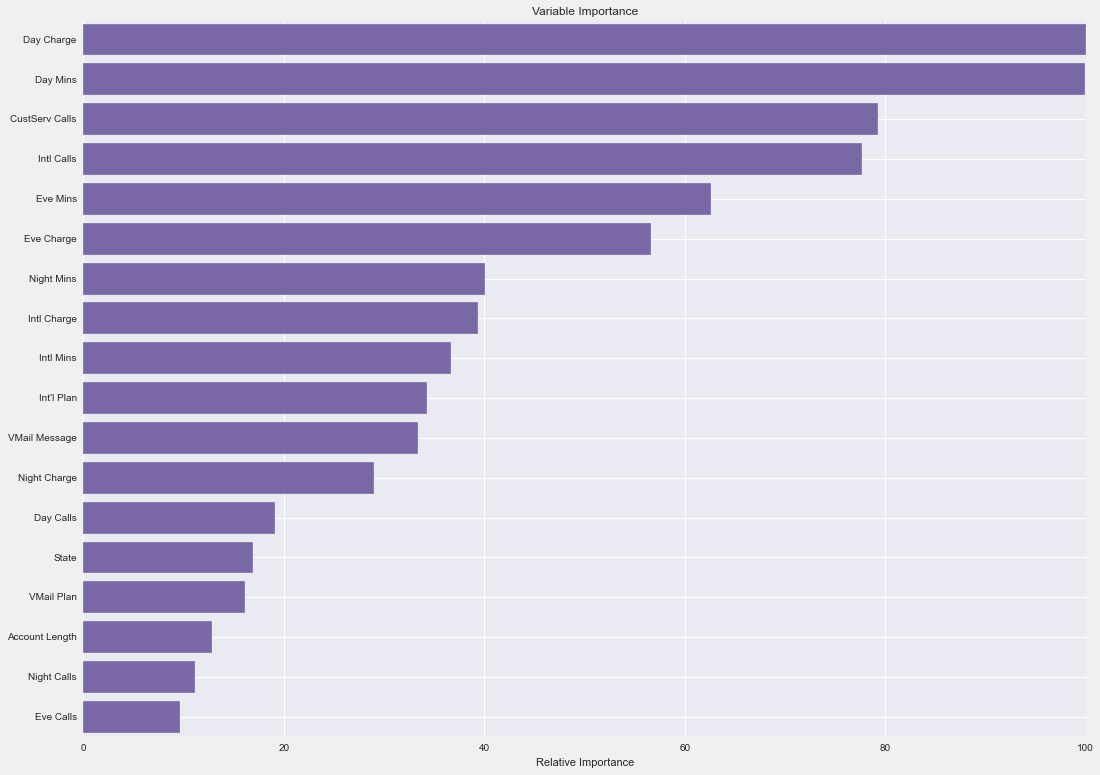### Polynomial Features¶

In :
X = df.as_matrix().astype(np.float)
polynomial_features = preprocessing.PolynomialFeatures()
X = polynomial_features.fit_transform(X)

In :
X.shape

Out:
(3333, 190)
In :
print('Passive Aggressive Classifier:\n {}\n'.format(metrics.classification_report(y, stratified_cv(X, y, linear_model.PassiveAggressiveClassifier))))
print('Gradient Boosting Classifier:\n {}\n'.format(metrics.classification_report(y, stratified_cv(X, y, ensemble.GradientBoostingClassifier))))
print('Support vector machine(SVM):\n {}\n'.format(metrics.classification_report(y, stratified_cv(X, y, svm.SVC))))
print('Random Forest Classifier:\n {}\n'.format(metrics.classification_report(y, stratified_cv(X, y, ensemble.RandomForestClassifier))))
print('K Nearest Neighbor Classifier:\n {}\n'.format(metrics.classification_report(y, stratified_cv(X, y, neighbors.KNeighborsClassifier))))
print('Logistic Regression:\n {}\n'.format(metrics.classification_report(y, stratified_cv(X, y, linear_model.LogisticRegression))))
print('Dump Classifier:\n {}\n'.format(metrics.classification_report(y, [0 for ii in y.tolist()]))); # ignore the warning as they are all 0

Passive Aggressive Classifier:
precision    recall  f1-score   support

0       0.86      1.00      0.92      2850
1       0.60      0.01      0.01       483

avg / total       0.82      0.86      0.79      3333

Gradient Boosting Classifier:
precision    recall  f1-score   support

0       0.96      0.99      0.98      2850
1       0.92      0.77      0.84       483

avg / total       0.96      0.96      0.96      3333

Support vector machine(SVM):
precision    recall  f1-score   support

0       0.86      1.00      0.92      2850
1       0.00      0.00      0.00       483

avg / total       0.73      0.86      0.79      3333

Random Forest Classifier:
precision    recall  f1-score   support

0       0.95      0.99      0.97      2850
1       0.92      0.71      0.80       483

avg / total       0.95      0.95      0.95      3333

K Nearest Neighbor Classifier:
precision    recall  f1-score   support

0       0.89      0.98      0.93      2850
1       0.68      0.31      0.43       483

avg / total       0.86      0.88      0.86      3333

Logistic Regression:
precision    recall  f1-score   support

0       0.92      0.97      0.95      2850
1       0.75      0.54      0.62       483

avg / total       0.90      0.91      0.90      3333

Dump Classifier:
precision    recall  f1-score   support

0       0.86      1.00      0.92      2850
1       0.00      0.00      0.00       483

avg / total       0.73      0.86      0.79      3333



### Scaled and Polynomial Features¶

If you think, when some of the features come together, they could form a much more powerful feature, or just by getting the square of the feature would be powerful feature, then Scikit-Learn has something that quite fits to your needs. Let's aasume I have [x, y] feature vector and I am interested in [1, x, y, x^2, xy, y^2], in the preprocessing step, I could use PolynomialFeatures of Scikit-Learn to build that feature matrix. If I just want to only get the interaction features(not x^2, then it is enough to pass interaction_only=True and include_bias=False. If you want to get higher order Polynomial features(say nth degree), pass degree=n optional parameter to Polynomial Features.

In :
X = df.as_matrix().astype(np.float)
scaler = preprocessing.StandardScaler()
X = scaler.fit_transform(X)
polynomial_features = preprocessing.PolynomialFeatures()
X = polynomial_features.fit_transform(X)

In :
print('Passive Aggressive Classifier:\n {}\n'.format(metrics.classification_report(y, stratified_cv(X, y, linear_model.PassiveAggressiveClassifier))))
print('Gradient Boosting Classifier:\n {}\n'.format(metrics.classification_report(y, stratified_cv(X, y, ensemble.GradientBoostingClassifier))))
print('Support vector machine(SVM):\n {}\n'.format(metrics.classification_report(y, stratified_cv(X, y, svm.SVC))))
print('Random Forest Classifier:\n {}\n'.format(metrics.classification_report(y, stratified_cv(X, y, ensemble.RandomForestClassifier))))
print('K Nearest Neighbor Classifier:\n {}\n'.format(metrics.classification_report(y, stratified_cv(X, y, neighbors.KNeighborsClassifier))))
print('Logistic Regression:\n {}\n'.format(metrics.classification_report(y, stratified_cv(X, y, linear_model.LogisticRegression))))
print('Dump Classifier:\n {}\n'.format(metrics.classification_report(y, [0 for ii in y.tolist()]))); # ignore the warning as they are all 0

Passive Aggressive Classifier:
precision    recall  f1-score   support

0       0.92      0.93      0.92      2850
1       0.56      0.52      0.54       483

avg / total       0.87      0.87      0.87      3333

Gradient Boosting Classifier:
precision    recall  f1-score   support

0       0.96      0.99      0.97      2850
1       0.92      0.75      0.82       483

avg / total       0.95      0.95      0.95      3333

Support vector machine(SVM):
precision    recall  f1-score   support

0       0.91      0.99      0.95      2850
1       0.92      0.43      0.58       483

avg / total       0.91      0.91      0.90      3333

Random Forest Classifier:
precision    recall  f1-score   support

0       0.94      0.99      0.97      2850
1       0.93      0.65      0.77       483

avg / total       0.94      0.94      0.94      3333

K Nearest Neighbor Classifier:
precision    recall  f1-score   support

0       0.89      0.99      0.94      2850
1       0.79      0.31      0.45       483

avg / total       0.88      0.89      0.87      3333

Logistic Regression:
precision    recall  f1-score   support

0       0.93      0.97      0.95      2850
1       0.76      0.55      0.64       483

avg / total       0.90      0.91      0.90      3333

Dump Classifier:
precision    recall  f1-score   support

0       0.86      1.00      0.92      2850
1       0.00      0.00      0.00       483

avg / total       0.73      0.86      0.79      3333



## Regression¶

Regresion refers learning how to relate the input variables to output variables. This is true for classification as well with only one difference that the regression would deal with continuous output variables where classification produces discrete output variables. One can use a regressor to output discrete variables as well using basic thresholding although it is not common.

In :
from sklearn.datasets.california_housing import fetch_california_housing

california_housing = sklearn.datasets.california_housing.fetch_california_housing()
california_housing_data = california_housing['data']
california_housing_labels = california_housing['target']# 'target' variables
california_housing_feature_names = california_housing['feature_names']

In :
california_housing_feature_names

Out:
['MedInc',
'HouseAge',
'AveRooms',
'AveBedrms',
'Population',
'AveOccup',
'Latitude',
'Longitude']

### Cross-Validation¶

• Split the data 80/20 rule, test_size parameter determines the ratio of test data in the whole dataset
In :
from sklearn import cross_validation
X_train, X_test, y_train, y_test = cross_validation.train_test_split(california_housing_data,
california_housing_labels,
test_size=0.2,
random_state=0)

In :
print(X_train.shape)
print(X_test.shape)
print('Training/Test Ratio: {}'.format(X_train.shape / X_test.shape))

(16512, 8)
(4128, 8)
Training/Test Ratio: 4


So far so good!

### Regression model parameters¶

In :
parameters = {
'n_estimators': 500,
'max_depth': 4,
'min_samples_split': 1,
'learning_rate': 0.01,
'loss': 'ls'
}


### We will use an ensemble model to predict housing price¶

In :
from sklearn import ensemble
from sklearn import metrics
classifier = ensemble.GradientBoostingRegressor(**parameters)

classifier.fit(X_train, y_train)
predictions = classifier.predict(X_test)
mse = metrics.mean_squared_error(y_test, predictions)
print('Mean Square Error: {:.3f}'.format(mse))

Mean Square Error: 0.295

In :
### This may take some time, but it will worth it I promise
parameters = {
'n_estimators': 3000,
'max_depth': 6,
'learning_rate': 0.04,
'loss': 'huber'
}
classifier = ensemble.GradientBoostingRegressor(**parameters)

classifier.fit(X_train, y_train)
predictions = classifier.predict(X_test)
mse = metrics.mean_squared_error(y_test, predictions)
print('Mean Squared Error: {:.3f}'.format(mse))

Mean Squared Error: 0.194


Yep, this is much better. But instead of setting the parameters like this and then look at the mean squared error, maybe I should use another way to optimize the parameters based on mean squared eror. I will see how to do that in the next section; GridSearch to optimize the parameters.

In :
plt.figure(figsize=(16, 12))

plt.scatter(range(predictions.shape), predictions, label='predictions', c='#348ABD', alpha=0.4)
plt.scatter(range(y_test.shape), y_test, label='actual values', c='#A60628', alpha=0.4)
plt.ylim([y_test.min(), predictions.max()])
plt.xlim([0, predictions.shape])
plt.legend();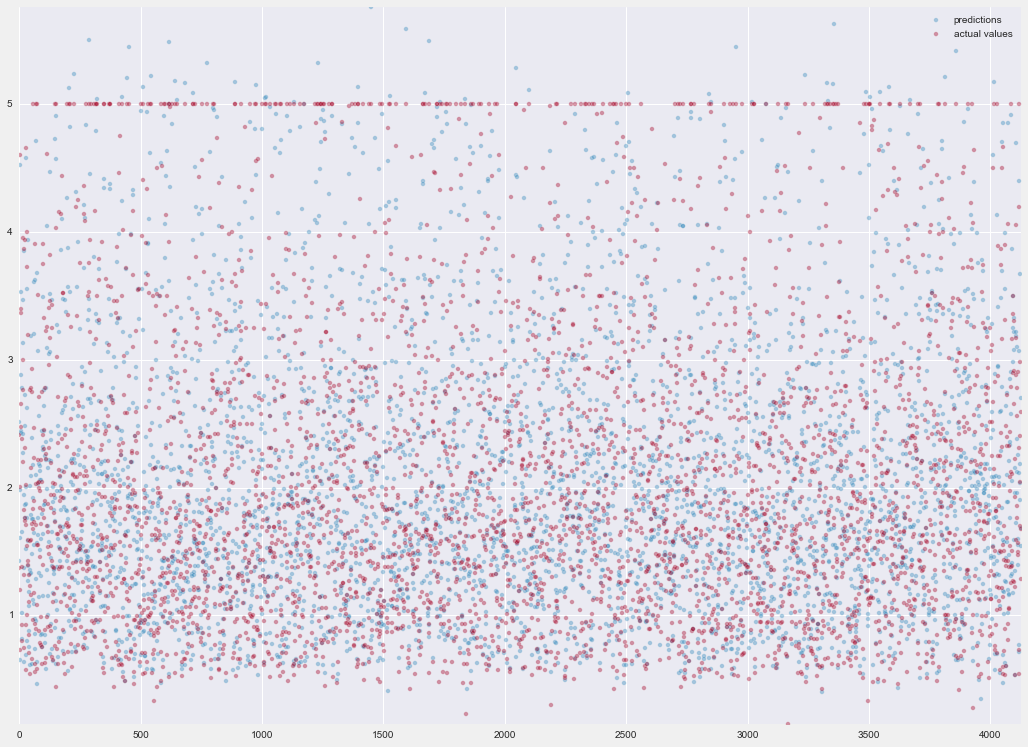In :
test_score = [classifier.loss_(y_test, y_pred) for y_pred in classifier.staged_decision_function(X_test)]

plt.figure(figsize=(16, 12))
plt.title('Deviance');
plt.plot(np.arange(parameters['n_estimators']) + 1, classifier.train_score_, c='#348ABD',
label='Training Set Deviance');
plt.plot(np.arange(parameters['n_estimators']) + 1, test_score, c='#A60628',
label='Test Set Deviance');
plt.annotate('Overfit Point', xy=(600, test_score), xycoords='data',
xytext=(420, 0.06), textcoords='data',
arrowprops=dict(arrowstyle="->", connectionstyle="arc"),
)
plt.legend(loc='upper right');
plt.xlabel('Boosting Iterations');
plt.ylabel('Deviance');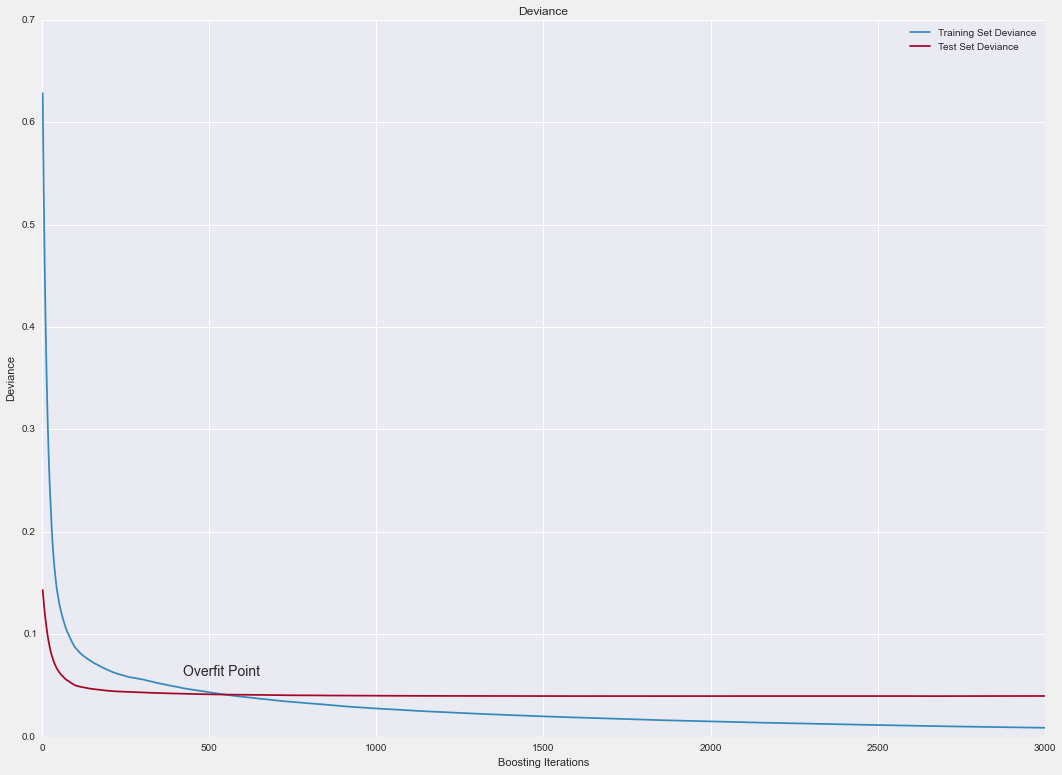The point that red line(test) is equal to blue line(training) is our sweet spot. We want to minimize the training error but also do not overfit. Test error stays same after 600-700 boosting iterations even if training error decreases up to 3000. Training error decreases because we are overfitting after 700 boosting iterations. That is also obvious that test error slightly increasee after 700. In general, both classification and also regression, in order to prevent overfitting, one not only looks at the success rate of the classifier/regressor in the training data but also in the test data as well.

In :
# Get Feature Importance from the classifier
feature_importance = classifier.feature_importances_
# Normalize The Features
feature_importance = 100.0 * (feature_importance / feature_importance.max())
sorted_idx = np.argsort(feature_importance)
pos = np.arange(sorted_idx.shape) + .5
plt.figure(figsize=(16, 12))
plt.barh(pos, feature_importance[sorted_idx], align='center', color='#7A68A6')
plt.yticks(pos, np.asanyarray(california_housing_feature_names)[sorted_idx])
plt.xlabel('Relative Importance')
plt.title('Variable Importance')
plt.show()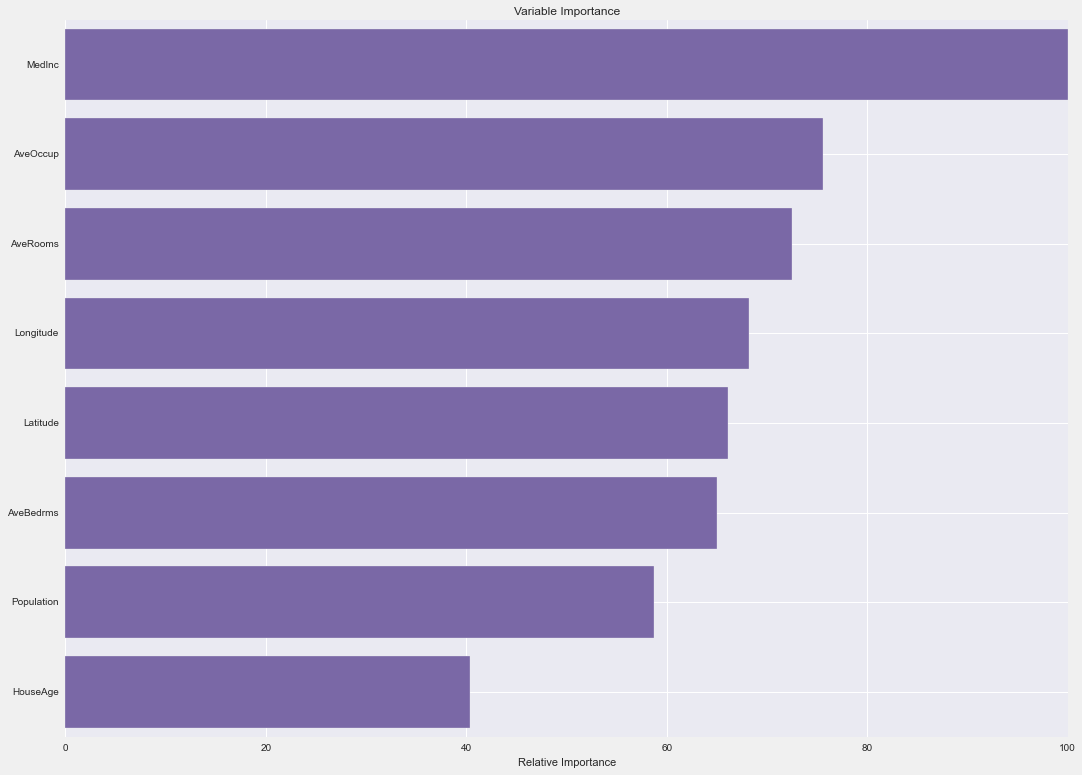In here I am plotting the relative importances of the features as RF could estimate which feature could play more important role than other features. This part generally is done in explaratory data analysis part but it is nice to have at least some idea on how classifier treats the features as well.

Classifiers both in terms of their architecture but also capabilities differ. If you do not know beforehand which feature is important to predict the outcome of the particular class, you may want to choose a classifier which has both feature selection capability(which could score the features based on how useful it is or minimizing least squared error like RandomForest does in this case). Some of the classifiers support class weighting(if one class is more important than the other one) through class_weight parameter (e.g.: Suppor Vector Classifier, SVC). When you want to choose a classifier, apart from its merits, these out of the box capabilities could also influence your thinking and make your decision about which classifier is best.

In :
features = ['MedInc', 'AveOccup', 'HouseAge', 'AveRooms',
('AveOccup', 'HouseAge')]
fig, ax = ensemble.partial_dependence.plot_partial_dependence(classifier, X_train, features,
feature_names=california_housing_feature_names,
figsize=(16, 12));

/Users/bugra/anaconda/lib/python2.7/site-packages/matplotlib/text.py:52: UnicodeWarning: Unicode equal comparison failed to convert both arguments to Unicode - interpreting them as being unequal
if rotation in ('horizontal', None):
/Users/bugra/anaconda/lib/python2.7/site-packages/matplotlib/text.py:54: UnicodeWarning: Unicode equal comparison failed to convert both arguments to Unicode - interpreting them as being unequal
elif rotation == 'vertical':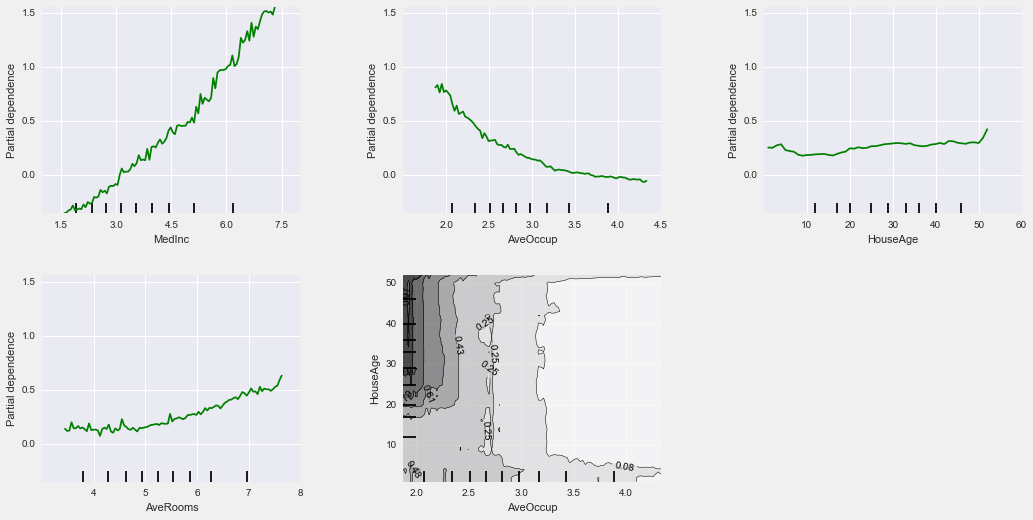In this part, one could also look at the partial dependence plots to see which feature influence more and also how tdoe they disribute across different feature categories.

I made an intentional mistake in this notebook.

• Can you think of what might go wrong in the feature set?
• Are features scaled? If not, can scaling help? Why?
• What type of scaling would be useful to preprocess the targer variable(price of house)? Why?
comments powered by Disqus